# Understanding of Convolutional Neural Network (CNN) — Deep Learning

In neural networks, Convolutional neural network (ConvNets or CNNs) is one of the main categories to do images recognition, images classifications. Objects detections, recognition faces etc., are some of the areas where CNNs are widely used.

CNN image classifications takes an input image, process it and classify it under certain categories (Eg., Dog, Cat, Tiger, Lion). Computers sees an input image as array of pixels and it depends on the image resolution. Based on the image resolution, it will see h x w x d( h = Height, w = Width, d = Dimension ). Eg., An image of 6 x 6 x 3 array of matrix of RGB (3 refers to RGB values) and an image of 4 x 4 x 1 array of matrix of grayscale image.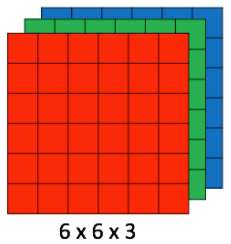Figure 1 : Array of RGB Matrix

Technically, deep learning CNN models to train and test, each input image will pass it through a series of convolution layers with filters (Kernals), Pooling, fully connected layers (FC) and apply Softmax function to classify an object with probabilistic values between 0 and 1. The below figure is a complete flow of CNN to process an input image and classifies the objects based on values.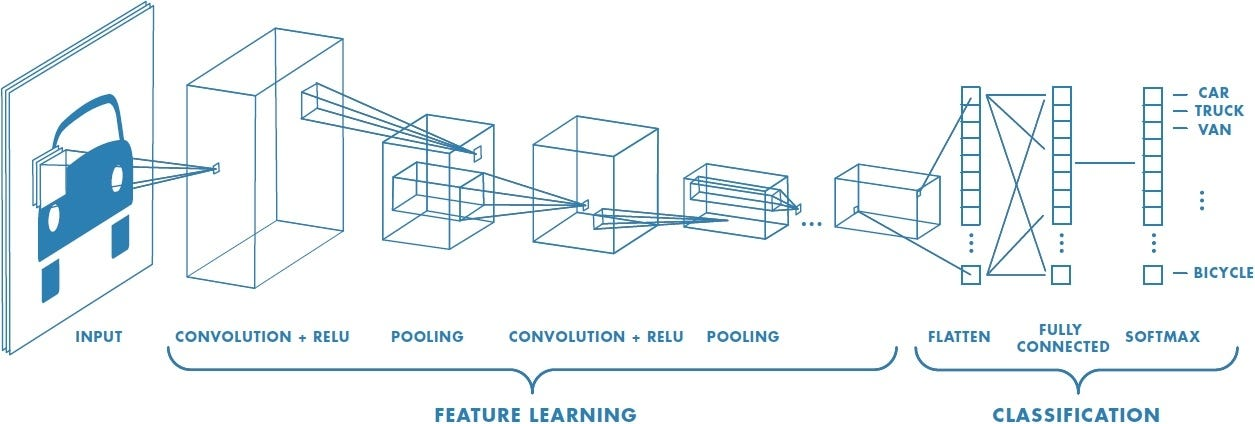Figure 2 : Neural network with many convolutional layers

Convolution Layer

Convolution is the first layer to extract features from an input image. Convolution preserves the relationship between pixels by learning image features using small squares of input data. It is a mathematical operation that takes two inputs such as image matrix and a filter or kernal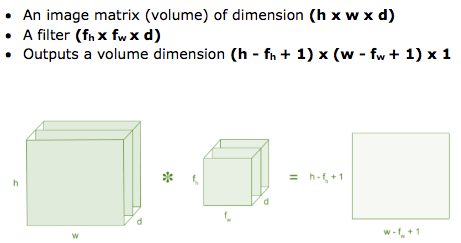Figure 3: Image matrix multiplies kernel or filter matrix

Consider a 5 x 5 whose image pixel values are 0, 1 and filter matrix 3 x 3 as shown in below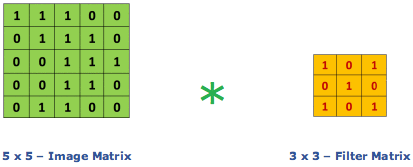Figure 4: Image matrix multiplies kernel or filter matrix

Then the convolution of 5 x 5 image matrix multiplies with 3 x 3 filter matrix which is called “Feature Map” as output shown in below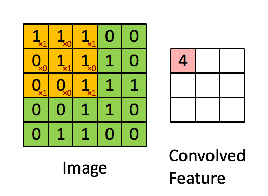Figure 5: 3 x 3 Output matrix

Convolution of an image with different filters can perform operations such as edge detection, blur and sharpen by applying filters. The below example shows various convolution image after applying different types of filters (Kernels).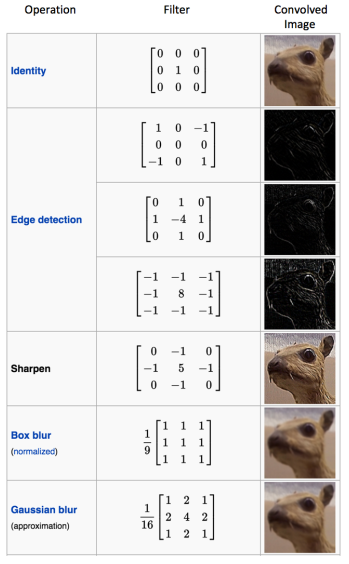Figure 7 : Some common filters

Strides

Stride is the number of pixels shifts over the input matrix. When the stride is 1 then we move the filters to 1 pixel at a time. When the stride is 2 then we move the filters to 2 pixels at a time and so on. The below figure shows convolution would work with a stride of 2.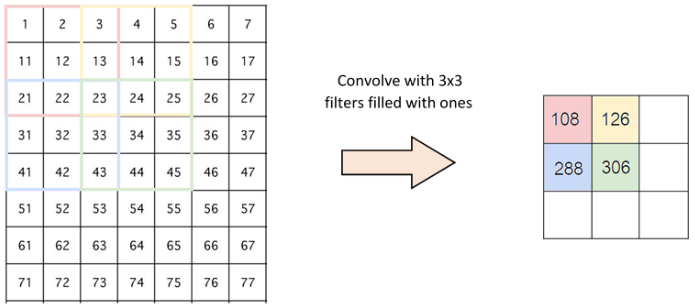Figure 6 : Stride of 2 pixels

Padding

Sometimes filter does not fit perfectly fit the input image. We have two options:

• Pad the picture with zeros (zero-padding) so that it fits
• Drop the part of the image where the filter did not fit. This is called valid padding which keeps only valid part of the image.

Non Linearity (ReLU)

ReLU stands for Rectified Linear Unit for a non-linear operation. The output is ƒ(x) = max(0,x).

Why ReLU is important : ReLU’s purpose is to introduce non-linearity in our ConvNet. Since, the real world data would want our ConvNet to learn would be non-negative linear values.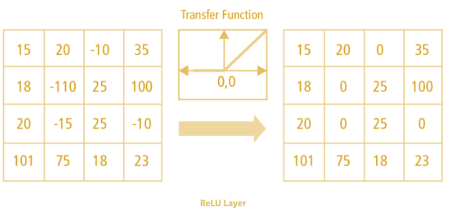Figure 7 : ReLU operation

There are other non linear functions such as tanh or sigmoid can also be used instead of ReLU. Most of the data scientists uses ReLU since performance wise ReLU is better than other two.

Pooling Layer

Pooling layers section would reduce the number of parameters when the images are too large. Spatial pooling also called subsampling or downsampling which reduces the dimensionality of each map but retains the important information. Spatial pooling can be of different types:

• Max Pooling
• Average Pooling
• Sum Pooling

Max pooling take the largest element from the rectified feature map. Taking the largest element could also take the average pooling. Sum of all elements in the feature map call as sum pooling.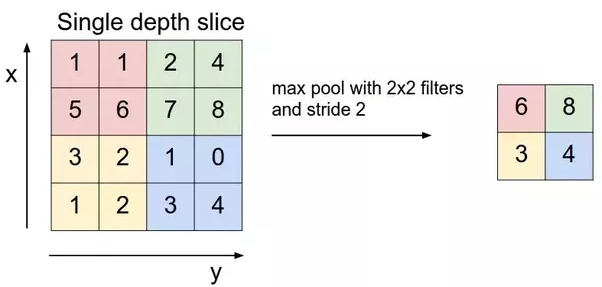Figure 8 : Max Pooling

Fully Connected Layer

The layer we call as FC layer, we flattened our matrix into vector and feed it into a fully connected layer like neural network.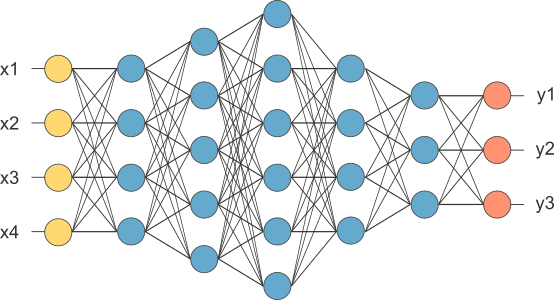Figure 9 : After pooling layer, flattened as FC layer

In the above diagram, feature map matrix will be converted as vector (x1, x2, x3, …). With the fully connected layers, we combined these features together to create a model. Finally, we have an activation function such as softmax or sigmoid to classify the outputs as cat, dog, car, truck etc.,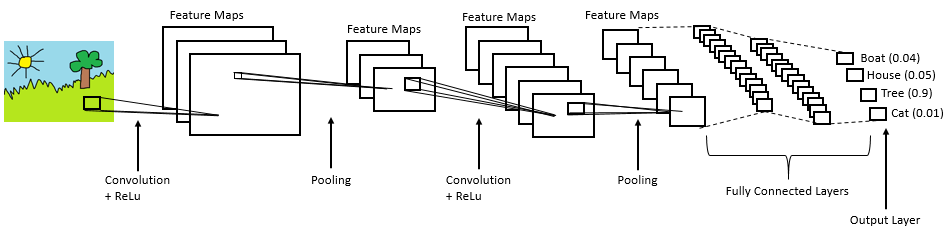Figure 10 : Complete CNN architecture

Summary

• Provide input image into convolution layer
• Choose parameters, apply filters with strides, padding if requires. Perform convolution on the image and apply ReLU activation to the matrix.
• Perform pooling to reduce dimensionality size
• Add as many convolutional layers until satisfied
• Flatten the output and feed into a fully connected layer (FC Layer)
• Output the class using an activation function (Logistic Regression with cost functions) and classifies images.

In the next post, I would like to talk about some popular CNN architectures such as AlexNet, VGGNet, GoogLeNet and ResNet.

References :